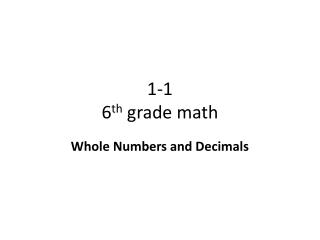DownloadDownload Presentation# 1-1 6 th grade math

Download Presentation## 1-1 6 th grade math

- - - - - - - - - - - - - - - - - - - - - - - - - - - E N D - - - - - - - - - - - - - - - - - - - - - - - - - - -
##### Presentation Transcript

1. 1-16th grade math Whole Numbers and Decimals

2. Objective • To use place value to understand whole numbers to the billions and decimals to the hundred-thousandths. • Why? To help you understand the value of all types of numbers

3. California State Standards NS 1.1 (Gr. 5): Manipulate very large (e.g., millions) and very small (e.g., thousandths) numbers. MR 1.0: Make decisions about how to approach problems.

4. Vocabulary • Base-ten numeration • Our number system is based on the Base-10 system. Numbers 1-9 are units. Add one more to 9 = 10 which translates to a new ‘house.’ • There can be all types of bases: Base 2 (binary, used in computer language), Base 5, etc. • Standard form • The way any numeral is written using only numbers 0-9 • 52,615,843 • Short word form - Using words and numbers to express a numeral - 52,615,843 = 52 million, 615 thousand, 843. • Place-value chart -Shows the order and names of the places for a number. - divided by ‘houses’: … billions, millions, thousands, one; decimals. Within in ‘house’, are rooms: thousands, ten, base.

5. Place Value Chart

6. How to Read Decimals To help you read decimal place values better, I have a little trick. Count the number of places in the decimal place value area. The amount of spaces you count tells you how many 0’s to have in your word. 52. 01. In the decimal place area, there are two place holders. The number with two 0’s is hundred. So, the number 52.01 is read 52 and 1 hundredths. 468. 0928. The decimal as four place holders. The number with four 0’s is ten-thousand. So the number is read 468 and 928 ten-thousandths.

7. How to Read Numbers with Decimals 1) In the whole numbers section, count the ‘houses’ and its ‘rooms’. 2) In the decimals section, count the number of spaces. The number of spaces = the number of 0’s to read the decimal number (ending with the suffix –ths). 3) Put it all together. Read the whole numbers first, then say the word AND then read the decimal. AND = the decimal. 6,035,479,518 = 6 billion, 35 million, 479 thousand, 518. 36.00025 There are five place holders in the decimal. The number hundred-thousand has five 0’s. = 36 and 25 hundred-thousandths 5.1267 = 5 and 1 thousand 2 hundred sixty-seven ten-thousandths.

8. Try It! Express the value in short work form. • 341,984,654 • 12,144,754,984 • 144.0086 • 23.00103 • Write this number: 625 ten-thousandths. • 4 ten millions • 2 billions • 6 ten-thousandths • 3 hundred-thousandths • 0._ _ _ _ = 0.0625

9. Objective Review • You can now use place value to understand whole numbers to the billions and decimals to the hundred-thousandths. • Why? You can now read larger numbers and smaller numbers (in decimals). This will help you later in your science classes: e.g., chemistry.

10. Independent Practice • Complete problems 5-16 • Copy original problem first. • Show all work! • If time, complete Mixed Review: 17-24 • If still more time, work on Accelerated Math.